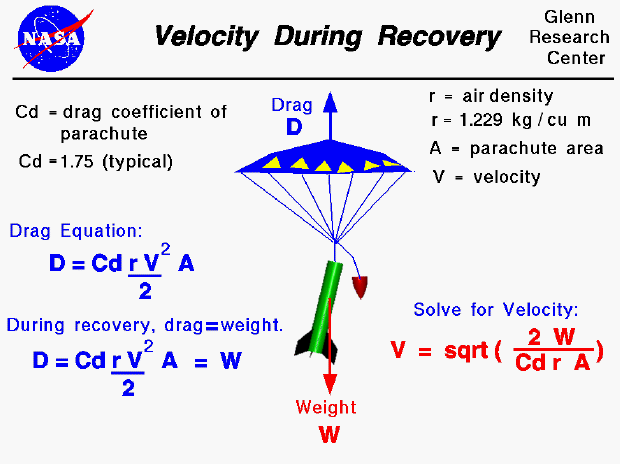The forces on a model rocket change dramatically in both magnitude and direction throughout a typical flight. This figure shows the velocity of a rocket during the recovery portion of the flight. At the end of the powered portion of the flight, the model rocket uses up all its fuel, the engine goes out and the thrust goes to zero. The rocket then coasts upward until the velocity goes to zero and the maximum altitude is reached. Because weight is still acting on the rocket, it immediately begins to fall back to earth. To slow the descent, an explosive charge is used to deploy a parachute. As the rocket falls, only two forces are acting on the rocket, the weight of the rocket and the aerodynamic drag on the parachute.

The weight (W) is always directed towards the center of the earth. By definition, the aerodynamic drag (D) is opposed to the flight direction. As the rocket descends, the drag and the weight are opposing forces. The vertical force (Fv) is the drag minus the weight.

Fv = D - W

The drag depends on the square of the velocity,. The rocket is falling at a high rate of speed when the parachute is deployed so the drag is high. The point is quickly reached where the drag is equal and opposite to the weight. At this point, there is no net external force on the rocket and from Newton's first law of motion the acceleration then becomes zero and the rocket falls at a constant terminal velocity.

We can determine the magnitude of the terminal velocity. As discussed above, the net vertical force on the rocket is zero and the drag is then equal to the weight. The drag equation states that the drag (D) is equal to some drag coefficient (Cd) times half of the air density (r) times the square of the velocity (V) times the reference area (A).

D = Cd * .5 * r * V^2 * A

We can equate this to the weight and solve for the velocity.

V = sqrt ( (2 * W) / (Cd * r * A) )

The terminal velocity depends on the weight, the drag coefficient, the air density and the reference area. The air density has a typical value of 1.229 kg/cu m. Typical values of drag coefficient for a parachute is about 1.75, compared to 0.75 for a typical model rocket. The higher drag coefficient produces a lower terminal velocity. The reference area is the large large cross-sectional area of the parachute which also produces a low terminal velocity. Compared to the descending rocket, the descent under parachute is slow and the rocket can survive the impact with the ground and fly again.

Guided Tours